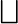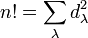# Robinson-Schensted correspondence

## Definition

Let$n$ be a nonnegative integer. The Robinson-Schensted correspondence for degree$n$ is a bijection:$S_n \leftrightarrow \bigsqcup (D_\lambda \times D_\lambda)$

where$S_n$ is the symmetric group of degree$n$ (taken to be permutations on$\{ 1,2,\dots, n\}$,$\lambda$ denotes an unordered integer partition of$n$, (or a Young diagram), and$D_\lambda$ is the set of all Young tableaux of shape$\lambda$. The disjoint union$\bigsqcup$ is carried out with$\lambda$ varying over the entire set of unordered integer partitions of$n$.

The bijection is not invariant under conjugation and heavily depends on the ordering properties on the set$\{ 1,2,\dots,n \}$.

Note: There are slightly different conventions that lead to different versions of the algorithm, so the exact bijection may differ in different descriptions of the correspondence.

### Description: permutation to tableau pair

We first provide an algorithm that takes as input a permutation$\sigma$ of$\{ 1,2,\dots,n \}$ expressed in one-line notation and outputs a pair of Young tableaux of the same shape. The two tableaux are called the position tableau and shape tableau. This algorithm is sometimes called a bumping algorithm and is described as follows:

• We read the permutation$\sigma$ in one-line notation from left to right. We begin with both the position tableau and shape tableau being empty, and for every letter we read, we make some change to both tableaux.
• Every time we read a letter, we compare it with the entries in the first column of the position tableau. If the letter we read is greater than the largest (bottom most) entry in the first column, we add it in a box at the bottom of the first column. Otherwise, we find the smallest entry in the first column bigger than it, and replace it with the incoming letter. That entry is now bumped off and we look for how to place it in the second column of the position tableau using the same procedure. We keep iterating this procedure until we have either placed the newly bumped letter at the bottom of a column or we run out of columns, in which case the newly bumped letter goes into a fresh column added at the right.
• Simultaneously, for the shape tableau, if we just read the$i^{th}$ letter from the left, we insert the box with label$i$ at the position where the position tableau had an actual new box added.

Note that:

• Both the position tableau and the shape tableau are tableaux.
• At every stage, the position and shape tableau have the same shape.
• Whereas the shape tableau has entries$1,2,\dots, i$, the position tableau has entries the first$i$ letters of the permutation being read so far. Thus, at the end, both have the entries$\{ 1,2,\dots,n \}$.

### Reverse algorithm: tableau pair to permutation

We carry out the bumping procedure in reverse.

## Illustration of bumping procedure

For a complete discussion with examples of every permutation on three elements, see Robinson-Schensted correspondence for symmetric group:S3.

## Significance of correspondence

If$d_\lambda$ denotes the cardinality of$D_\lambda$, i.e., the number of Young tableaux with shape a given Young diagram$\lambda$, then we have:$n! = \sum_{\lambda} d_\lambda^2$

This confirms something that we know/can deduce through another approach: for the symmetric group of degree$n$, the irreducible representations are parameterized by the set of unordered integer partitions, and the degree of the representation parameterized by a particular partition$\lambda$ equals$d_\lambda$ (which can be computed using the hook length formula). Thus, the above fact is consistent with the fact that sum of squares of degrees of irreducible representations equals group order.